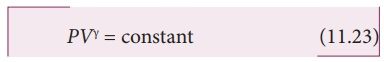Home | | Physics 11th std | Laplace’s correction - Propagation of Sound Waves

# Laplace’s correction - Propagation of Sound Waves

the exchange of heat produced due to compression and cooling effect due to rarefaction do not take place, because, air (medium) is a bad conductor of heat.

Laplace’s correction

In 1816, Laplace satisfactorily corrected this discrepancy by assuming that when the sound propagates through a medium, the particles oscillate very rapidly such that the compression and rarefaction occur very fast. Hence the exchange of heat produced due to compression and cooling effect due to rarefaction do not take place, because, air (medium) is a bad conductor of heat. Since, temperature is no longer considered as a constant here, sound propagation is an adiabatic process. By adiabatic considerations, the gas obeys Poisson’s law (not Boyle’s law as Newton assumed), which iswhich is the ratio between specific heat at constant pressure and specific heat at constant volume.

Differentiating equation (11.23) on both the sides, we getwhere, BA is the adiabatic bulk modulus of air. Now, substituting equation (11.24) in equation (11.16), the speed of sound in air isSince air contains mainly, nitrogen, oxygen, hydrogen etc, (diatomic gas), we take γ= 1.47. Hence, speed of sound in air is vA = ( √1.4)(280 m s-1)= 331.30 m s-1, which is very much closer to experimental data.

Study Material, Lecturing Notes, Assignment, Reference, Wiki description explanation, brief detail
11th Physics : UNIT 11 : Waves : Laplace’s correction - Propagation of Sound Waves |